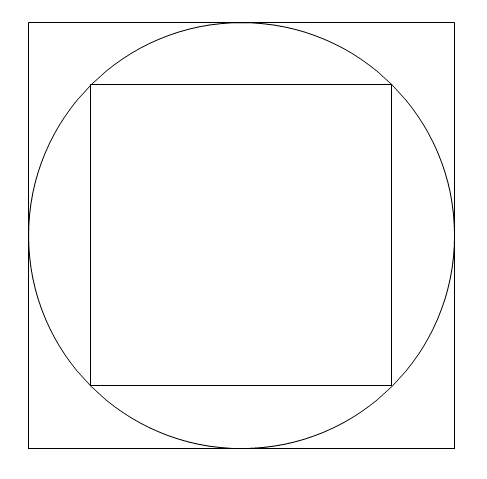# Thread: Area of Square in Circle

1. ## Area of Square in Circle

There is a shaded square inside a circle represented by the equation x^2 + y^2 = 9. Find the area of the square in the circle.

I seek the first-two steps.

2. ## Re: Area of Square in Circle

I am assuming the square is inscribed within the circle, and so the diagonal of the square is equal to the diameter of the circle.

Let $\displaystyle D=2r$ by the diameter of the circle. The area $\displaystyle A$ of the square is:

$\displaystyle A=s^2$

And we know:

$\displaystyle 2r=\sqrt{2}s\implies s=\sqrt{2}r\implies A=2r^2$

Can you proceed?

3. ## Re: Area of Square in CircleOriginally Posted by MarkFLI am assuming the square is inscribed within the circle, and so the diagonal of the square is equal to the diameter of the circle.

Let $\displaystyle D=2r$ by the diameter of the circle. The area $\displaystyle A$ of the square is:

$\displaystyle A=s^2$

And we know:

$\displaystyle 2r=\sqrt{2}s\implies s=\sqrt{2}r\implies A=2r^2$

Can you proceed?
A = 2r^2

I know that x^2 + y^2 = r^2.

So, r^2 = 9 leading to an r value of 3.

A = 2r^2

A = 2(3)^2

A = 2(9)

A = 18 units^2

4. ## Re: Area of Square in Circle

What would the area of a square be that circumscribes a circle of radius $\displaystyle r$? (This is there the circle is inside the square)

5. ## Re: Area of Square in CircleOriginally Posted by MarkFLWhat would the area of a square be that circumscribes a circle of radius $\displaystyle r$? (This is there the circle is inside the square)
If I know r, then A = 2r^2 yields the area of a square inside a circle.

6. ## Re: Area of Square in CircleOriginally Posted by harpazoIf I know r, then A = 2r^2 yields the area of a square inside a circle.
Rethink that! Are you missing some brackets?

7. ## Re: Area of Square in CircleOriginally Posted by DebstaRethink that! Are you missing some brackets?
A = 2(r)^2

8. ## Re: Area of Square in CircleOriginally Posted by harpazoIf I know r, then A = 2r^2 yields the area of a square inside a circle.
If the circle is inscribed within the square, then the side length of the square will be equal to the diameter of the circle, thus:

$\displaystyle A=(2r)^2=4r^2$

Now, given the result for the original problem you posted, we now know:

$\displaystyle 2r^2<\pi r^2<4r^2$

Or:

$\displaystyle 2<\pi<4$

How could we improve these bounds on $\displaystyle \pi$?

9. ## Re: Area of Square in CircleOriginally Posted by MarkFLIf the circle is inscribed within the square, then the side length of the square will be equal to the diameter of the circle, thus:

$\displaystyle A=(2r)^2=4r^2$

Now, given the result for the original problem you posted, we now know:

$\displaystyle 2r^2<\pi r^2<4r^2$

Or:

$\displaystyle 2<\pi<4$

How could we improve these bounds on $\displaystyle \pi$?
Sorry but I don't follow? Is my answer to the original question right?

10. ## Re: Area of Square in CircleOriginally Posted by harpazoSorry but I don't follow? Is my answer to the original question right?
Yes, you answered the original problem correctly, and is why I thanked your post.

Consider the following diagram:Can you see that the side length of the larger square is equal to the diameter of the circle inscribed within?

11. ## Re: Area of Square in CircleOriginally Posted by MarkFLYes, you answered the original problem correctly, and is why I thanked your post.

Consider the following diagram:Can you see that the side length of the larger square is equal to the diameter of the circle inscribed within?
Yes, I can what you are saying here.

12. ## Re: Area of Square in Circle

Do you see how the rest of what I posted in post #8 then follows?

13. ## Re: Area of Square in CircleOriginally Posted by MarkFLDo you see how the rest of what I posted in post #8 then follows?
Yes.

14. ## Re: Area of Square in CircleOriginally Posted by harpazoYes.
Okay, then can you think of a way we could improve our bounds on the value of pi?

15. ## Re: Area of Square in CircleOriginally Posted by MarkFLOkay, then can you think of a way we could improve our bounds on the value of pi?
Improve our bounds???